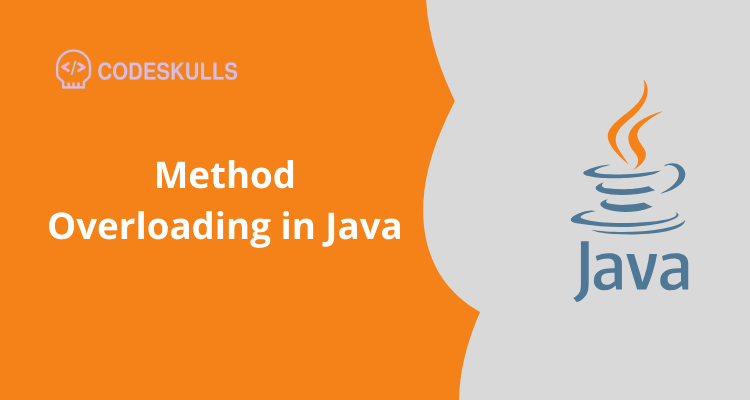Method overloading is one of the features of OOP if there is a class with the same name with a different number of parameters or different data types

## Number of parameters#

For overloading a method, two methods can have same name but , number of parameters differ

method(int, int)
method(int, int, int)

Example

return a+b;
}
static int add(int a,int b,int c){
return a+b+c;
}
}
public static void main(String[] args){
}
}
Output30
159

In the above example add( ) method is overloaded with respective of number of parameters.so if there is two arguments the first method will be executed add(int a,int b) , if there is there is three arguments the second method will be executed add(int a,int b,int c)

## Data type of parameters.#

For overloading a method, two methods can have same name but , number of parameters should be same and Data type of parameters differ

method(int, int)
method(int, float)

Example

return a+b;
}
return a+b;
}
}
public static void main(String[] args){
}
}
Output30
118.80000000000001

In the above example add( ) method is overloaded with respective of Data type of parameters. so if there is two arguments which have int as the datatype. the first method will be executed add(int a,int b)

so if there is two arguments which has double as the datatype . the second method will be executed add(double a,double b)

### Why Method Overloading is not possible by changing the return type of method?#

It is not possible to decide to execute which method based on the return type. This is called ambiguity.

Last updated on by vishal devxo